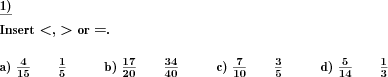Custom math worksheets at your fingertips# Details for problem "Compare two fractions and tell which one is larger"

Quickname: 1433

Elementary School, Primary School, Junior High School, Middle School, High School.

## Summary

Insert the correct relational symbol between two fractions

## Example## Description

Two fractions are given. The correct relational symbol has to be chosen and inserted in a blank space between the fractions. The larger and the smaller fraction have to be determined.

The number of problems and the number range for the numerators and denominators can be specified.

As a simplification, the numerators or denominators of both fractions can be chosen to be equal.

Download free printable worksheets for this math problem here. The worksheet contains the problems only, the solution sheet includes the answers. Just click on the respective link.

•Worksheet 1Solution sheet with answers
•Worksheet 2Solution sheet with answers
•Worksheet 3Solution sheet with answers

If you can not see the solution sheets for download, they may be filtered out by an ad blocker that you may have installed. If this is the case, please allow ads for this page and reload the page. The solution sheets will then reappear.

• Do these sample worksheets do not really fit?
• Do you need more math worksheets, with a different level of difficulty?
• Would you like to combine different problems on a worksheet and adjust them to your needs?
• As a teacher, you can put together your own worksheets using the automatically generated math problems provided.
With a free initial credit, you can start creating your own math worksheets in a few minutes.

You can try it for free! Register here, to create custom worksheets now!

## Customization options for this problem

Parameter
Possible values
Number of problems
1, 2, 3, 4, 5, 6, 7, 8, 9, 10
Number range
20, 30, 40, 50, 100, 200, 500, 1000, 10000, 100000
Simplification
None, Same denominator, Same numerator

## Similar problems

Remark
Description
Similar, with decimal fractions
Insert the correct relational symbol between two decimals.
Ordering more than two fractions: Put a series of fractions in the correct order
A series of fractions has to be put in the correct order.